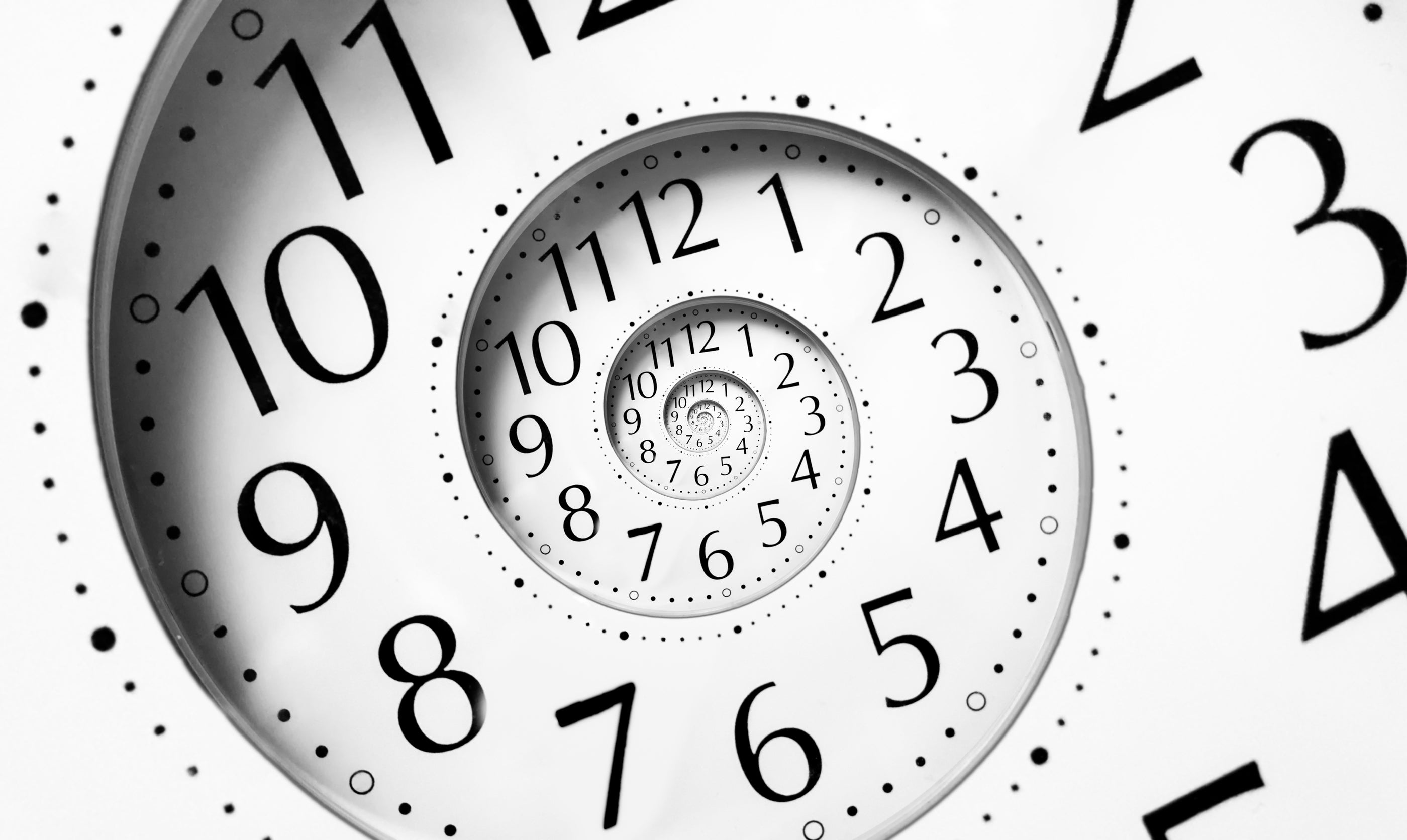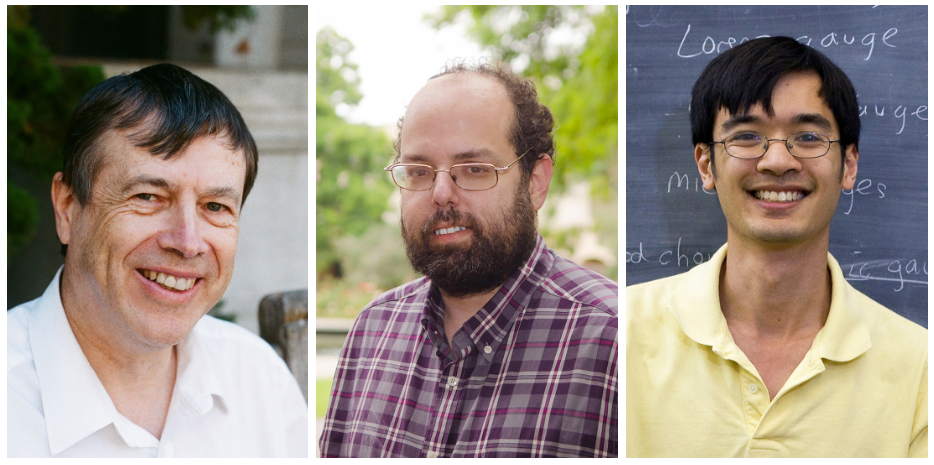# Smaller Is Better: Why Finite Number Systems Pack More Punch

Recent progress on the “sum product” problem recalls a celebrated mathematical result that revealed the power of miniature number systems.Explore

It’s one thing to turn a cartwheel in an open field. It’s another to manage it in a tight space like a bathtub. And that, in a way, is the spirit of one of the most important results in number theory over the past two decades.

The result has to do with the “sum-product problem,” which I wrote about last week. It asks you to take any set of numbers and arrange them in a square grid, then fill in the grid with either the sums or the products of the crosswise pairs.

The sum-product problem states that the number of distinct sums or products will always be close to N2 (where N stands for the number of numbers used to make your grid).

The sum-product problem that I wrote about uses any set of real numbers to generate the grid. You can also restrict the problem to use unique number systems that are smaller and more constrained than the reals. These self-contained number systems are called “finite fields.”

In mathematics, a “field” is any number system in which you can carry out the four basic operations of arithmetic: addition, subtraction, multiplication, and division. The real numbers form a field. You can perform those operations on any two real numbers and the outcome is a third real number. Or, put another way, the arithmetic of the real numbers never yields a number outside the real numbers.

The integers—all the positive and negative counting numbers—don’t form a field. Yes, you can add, subtract and multiply any two integers to produce a third integer. But divide 3 by 2 and you’ll get 1½, which isn’t an integer.

A “finite” field is a number system in which the number of numbers is finite. There are different kinds of finite fields, but the simplest one has to do with what’s called “modular” or “clock” arithmetic. In modular arithmetic, when you get to the end of your finite list of numbers, you just loop back to the beginning, as if you were counting numbers around the face of a clock. For example, if you go to a party at 7 p.m. and get back home six hours later, you’ll return at 1 a.m. More formally, 7 plus 6 in the base-12 modular number system equals 1.

(The 12 numbers on the clock don’t actually form a field, and the reason why has to do with one of the most critical features of number theory: Modular number systems form fields only when they’re comprised of a prime number of elements. In modular number systems with a nonprime number of elements, like the 12-digit clock, you end up in weird situations where the product of two nonzero numbers is zero. For example, 6 × 4 = 24, which equals zero in the base-12 number system. This leads to other consequences that cause division to break down. But in a modular number system with a prime number of elements, two nonzero numbers never multiply to zero.)

Finite fields have been the setting for many celebrated results in mathematics. As self-contained arithmetic worlds, they contain a rich structure that mathematicians can exploit to solve problems related to everything from prime numbers to patterns in the solutions to polynomial equations.

In 2003, the mathematicians Jean BourgainNets Katz, and Terry Tao became the first mathematicians to make any progress on the sum-product problem over finite fields. They proved that either the number of distinct sums or the number of distinct products must be at least ever-so-slightly larger than the size of the set used to generate the sum and product grids. The statement was modest in size but momentous in significance.Jean Bourgain, Nets Katz, and Terry Tao (from left) proved a milestone connection between addition and multiplication.George M. Bergman, Berkeley (Bourgain); Caltech (Katz); Reed Hutchinson/UCLA (Tao)

“It was the slightest thing we could get, but the point of it was it was the first result of its kind,” said Katz, who is now at the California Institute of Technology.

The authors of the paper were a powerhouse team: Katz is a highly respected number theorist, and Bourgain and Tao are regarded as among the top mathematicians of their generations. Bourgain, who died of cancer in December at the age of 64, was the driving force behind the proof. Several years previously he’d solved a different kind of sum-product problem. When he turned to the finite-field version, he had a fairly clear idea of how to pursue a proof, but he brought in Katz and Tao for help understanding all the implications of his intended method.

“Basically Bourgain knew how to do it and wanted our help because he wanted to write out some of the applications of [his approach],” said Katz.

Since 2003 other mathematicians have improved on the trio’s result by establishing that the number of distinct sums or products has to be even larger than what those three were able to guarantee. Mathematicians have also applied techniques from their proof to entirely different questions in math, including the study of objects called expander graphs and questions about polynomials and prime numbers.

Finite fields, which you can hold in your hand, might seem to be a less ambitious setting for the sum-product problem than the real numbers. But in fact, the question has much greater depth in the finite-field case, and far more implications for the rest of mathematics.

The reason is that it’s much harder for the sum-product phenomenon to be true for finite fields than for real numbers. The original formulation of the problem predicted that any set of numbers will produce sum and product grids with many more distinct entries than the size of the set itself. Perhaps that’s not a stunning statement when considered among the real numbers, which go on forever. But to be true in finite fields, where there’s barely any room to move? That’s like pulling off a cartwheel in a bathtub.

“The reals are an infinite set, and there’s lots and lots of room to grow. But in a finite field there’s only a very limited space to grow, so when you get a guarantee that some of that growth will happen, it’s kind of a stronger statement,” said Katz.

Lead image: Clock arithmetic describes any finite number system that loops back upon itself. Credit: liseykinaEnjoy unlimited Nautilus articles, ad-free, for as little as \$4.92/month.